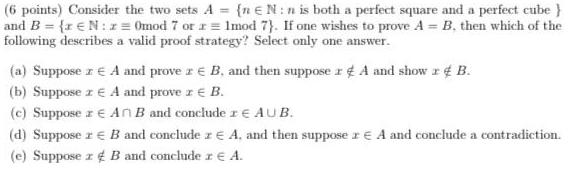Question:

# Consider the two sets A = {n EN:n is both a perfect square

Last updated: 8/14/2022Consider the two sets A = {n EN:n is both a perfect square and a perfect cube} and B = {r € N:r = 0mod 7 or r = 1mod 7). If one wishes to prove A = B. then which of the following describes a valid proof strategy? Select only one answer. (a) Suppose z € A and prove r e B, and then suppose r&A and show r & B. (b) Suppose z E A and prove z € B. (c) Suppose z € An B and conclude re AUB. (d) Suppose z € B and conclude z E A. and then suppose z E A and conclude a contradiction. (e) Suppose z B and conclude z € A.